# Problem 57998. Easy Sequences 111: Repnums as Hypotenuse of Pythagorean Triangles

The number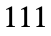belongs to a class of numbers called Repunit Numbers, in which all digits are 1.
For this problem, we'll define a superset of the repunit numbers which we shall call repnums, which are composed of repeated numbers. That is, if we denote repnums by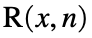, then x is the number to be repeated and n is the number of repetitions. Hence,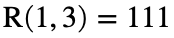,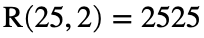,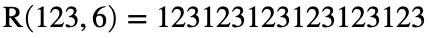and so on.
We can see from the figure below that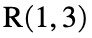can be a hypotenuse of a right triangle with integer sides (Pythagorean Triangle).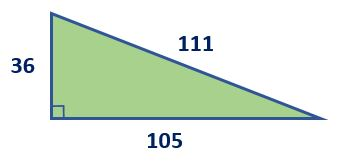In fact, this is the only Pythagorean triangle that can be formed with hypotenuse equal to. There is also only one Pythagorean triangle with hypotenuse equal to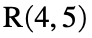, that is the triangle with sides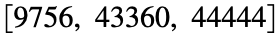, while there are seven Pythagorean triangles with hypotenures of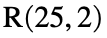, with legs as follows: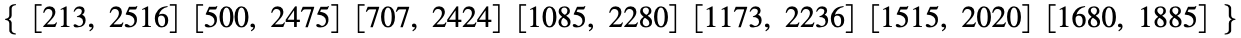Create the function,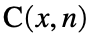, that counts the number of Pythagorean triangles that can be formed with hypotenuse equal to.

### Solution Stats

100.0% Correct | 0.0% Incorrect
Last Solution submitted on Jul 01, 2023

### Community Treasure Hunt

Find the treasures in MATLAB Central and discover how the community can help you!

Start Hunting!